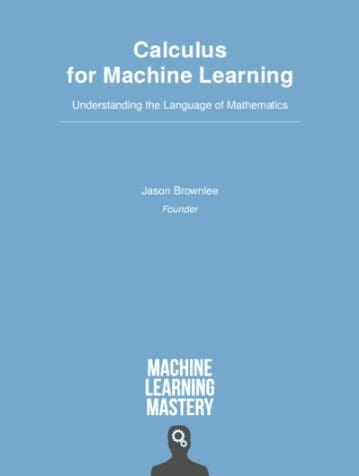# Calculus: The hidden building block of machine learning

Unless you have a basic knowledge of calculus, you cannot understand how machine learning algorithms are developed. Calculus for Machine Learning is designed for developers to get you up to speed on the calculus that you need for applied machine learning. The book has more math than our other books and over 85 code examples to help you understand the concepts.

Did you know calculus is the hidden building block of machine learning?

Machine learning benefits greatly from calculus. The gradient in gradient descent is indeed a calculus term.

Bootstrap the math and symbols with our new book:

>> Calculus for Machine Learning
(use the offer code 20offearlybird to get 20% off)Developers don't know calculus, and it’s a huge problem.

Unless you have a basic knowledge of calculus, you cannot understand how machine learning algorithms are developed.

For example, you need to know:

• How to find the gradient of the function to optimize
• How to use chain rule in backpropagation algorithm
• Understand how to find the solution to linear regression using least square estimator

The biggest mistake that beginners make when trying to learn calculus is that they try to learn the whole field, like an undergraduate university student:

• ...they study the wrong methods (e.g., not used in projects).
• ...they study the wrong way (e.g., theory first).

I have developed a playbook titled Calculus for Machine Learning designed for developers to get you up to speed on the calculus that you need for applied machine learning. The book has more math than our other books and over 85 code examples to help you understand the concepts.

It covers:

• Only the topics that are most relevant to machine learning.
• Everything is explained with results verifiable by Python code examples.
• There are no proofs or minimal derivations and it is always connected to practical use.

>> Click to get the calculus you need for machine learning

As a valued reader, I want to give you a discount on this new book.

Enter the offer code 20offearlybird and click "apply" to get 20% off the price of the standalone book.

I'll speak to you soon.

Jason,
Founder, Machine Learning Mastery